# #先锋团#卡尔曼滤波及其变种有哪些？究竟滤了个啥？ 原创 精华

0收藏

## 从基础卡尔曼滤波到互补卡尔曼滤波

1. 基础卡尔曼滤波——对线性系统的预测
2. 扩展卡尔曼滤波——基础卡尔曼滤波在非线性系统的扩展
3. 基于四元素的卡尔曼滤波器——基于实际问题的讲解
4. 状态误差卡尔曼滤波——将状态误差引入状态向量
5. 互补卡尔曼滤波——一种只使用角度误差和角速度误差作为状态向量和测量向量的滤波器形式

## 基础卡尔曼滤波器

### 宏观认识

1. 预测（prediction）—— Dynamic model
2. 更新（correction/measurment update）—— Observation model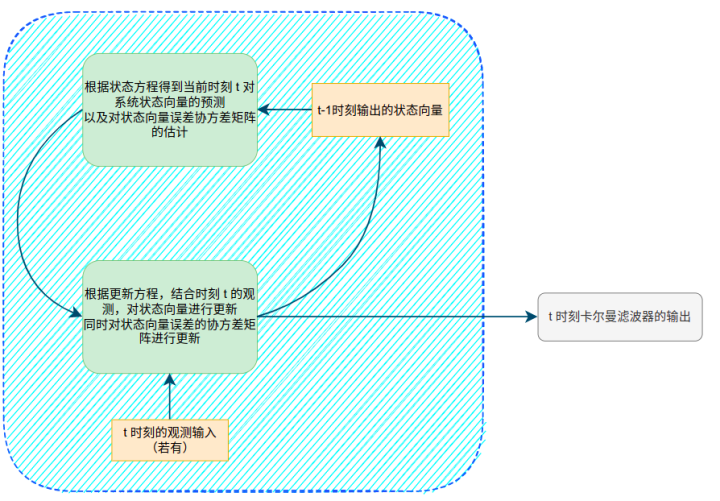### 状态方程及测量方程

$$\mathbf{x}t = A_t\mathbf{x}{t-1}+B_t\mathbf{u}_t+\mathbf{w}_t\tag{1-1}$$
$$\mathbf{z}_t = H\mathbf{x}_t+\mathbf{v}_t\tag{1-2}$$

$$\mathbf{w}_t\sim N(0,Q_t)$$
$$\mathbf{v}_t\sim N(0,R_t)$$

### 预测过程

$$\hat{\mathbf{x}}t^-=A_t\hat{\mathbf{x}}{t-1}^++B_t\mathbf{u}_t\tag{1-3}$$

$$P_t^-=A_tP_{t-1}^+A_t^T+Q_t\tag{1-4}$$

$$P_t^-\triangleq \mathbb{E}[(\mathbf{x}_t-\hat{\mathbf{x}}_t^-)(\mathbf{x}_t-\hat{\mathbf{x}}_t^-)^T]$$

### 更新过程

$$\tilde{\mathbf{y}}_t=\mathbf{z}_t-H_t\hat{\mathbf{x}}_t^-$$

$$S_t=H_tP_t^-H_t^T+R_t$$

$$K_t=P_t^-H_t^TS_t^{-1}\tag{1-5}$$

$$\mathbf{x}_t^+=\mathbf{x}_t^-+K_t\tilde{\mathbf{y}}_t\tag{1-6}$$

$$P_t^+=(I-K_tH_t)P_t^-\tag{1-7}$$

### 详细公式推导

1. 卡尔曼滤波基础知识及公式推导——较为形象化地讲解预测和更新这两个过程之间地概率分布关系
2. wiki Kalman Filter——准确的公式化推导

### 如何理解卡尔曼滤波器？

#### 从最小化误差的角度

$$\mathbf{e}_t = \mathbf{x}_t - \hat{\mathbf{x}}_t$$

## 扩展卡尔曼滤波

### 非线性方程的线性近似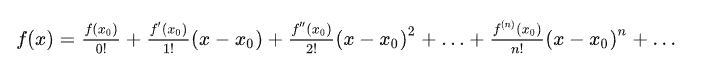### 状态方程及测量方程

$$\mathbf{x}t=f(\mathbf{x}{t-1}, \mathbf{u}_t, \mathbf{w}_t)\tag{2-1}$$

$$\mathbf{z}_t=h(\mathbf{x}_t,\mathbf{v}_t)\tag{2-2}$$

### 一阶泰勒展开

$$\hat{\mathbf{x}}{t-1}^+,P{t-1}^+$$

$$\hat{\mathbf{x}}t^-=f(\hat{\mathbf{x}}{t-1}^+, \mathbf{u}_t, 0)\tag{2-3}$$

$$\mathbf{x}t\approx \hat{\mathbf{x}}t^-+A_t(\mathbf{x}{t-1}-\hat{\mathbf{x}}{t-1}^+)+W_t\mathbf{w}_t\tag{2-4}$$

$$\mathbf{z}_t\approx \hat{\mathbf{z}}_t^-+H(\mathbf{x}_t-\hat{\mathbf{x}}_t^-)+V_t\mathbf{v}_t\tag{2-5}$$

• $A_{[i,j]}=\frac{\partial f_{i}}{\partial x_j}(\hat{\mathbf{x}}_{t-1}^+, \mathbf{u}_t, 0)$
• $W_{[i,j]}=\frac{\partial f_i}{\partial w_j}(\hat{\mathbf{x}}_{t-1}^+, \mathbf{u}_t, 0)$
• $H_{[i,j]}=\frac{\partial h_i}{\partial x_j}(\hat{\mathbf{x}}_t^-,0)$
• $V_{[i,j]}=\frac{\partial h_i}{\partial v_j}(\hat{\mathbf{x}}_t^-,0)$

### 预测方程及状态协方差矩阵

$$\hat{\mathbf{x}}t^-=f(\hat{\mathbf{x}}{t-1}^+,\mathbf{u}t,0)\tag{2-6}$$
$$P_t^-=A_tP {t-1}^-A_t^T+W_tQ_tW_t^T\tag{2-7}$$

$$W_t\mathbf{w}_t\sim N(0, W_tQW_t^T)$$

### 更新方程及卡尔曼增益

$$\tilde{\mathbf{y}}_t=\mathbf{z}_t-h(\hat{\mathbf{x}}_t^-,0)$$
$$S_t=H_tP_t^-H_t^T+V_tR_tV_t^T$$
$$K_t=P_t^-H_t^TS_t^{-1}\tag{2-8}$$
$$\hat{x}_t^+=\hat{\mathbf{x}}_t^-+K_t\tilde{\mathbf{y}}_t\tag{2-9}$$
$$P_t^+=(I-K_tH_t)P_t^-\tag{2-10}$$

## 基础卡尔曼滤波器 && 扩展卡尔曼滤波器总结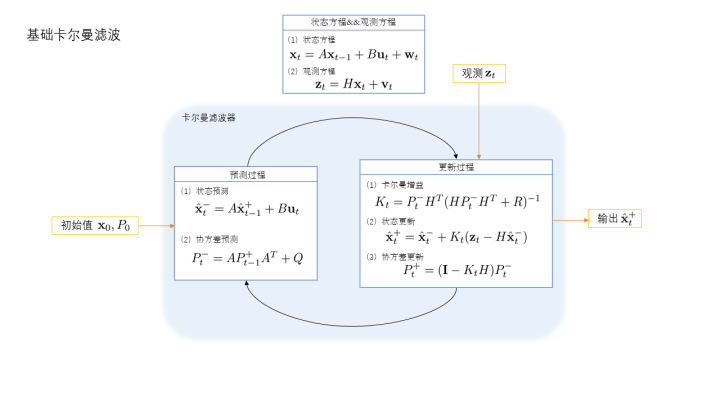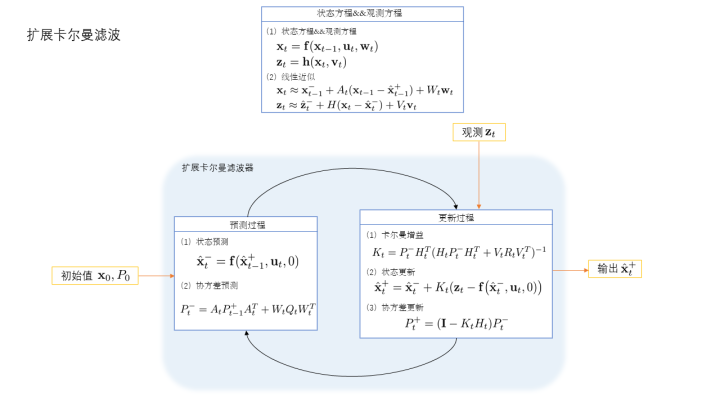## 基于四元数的拓展卡尔曼滤波器

### 从实际问题讲起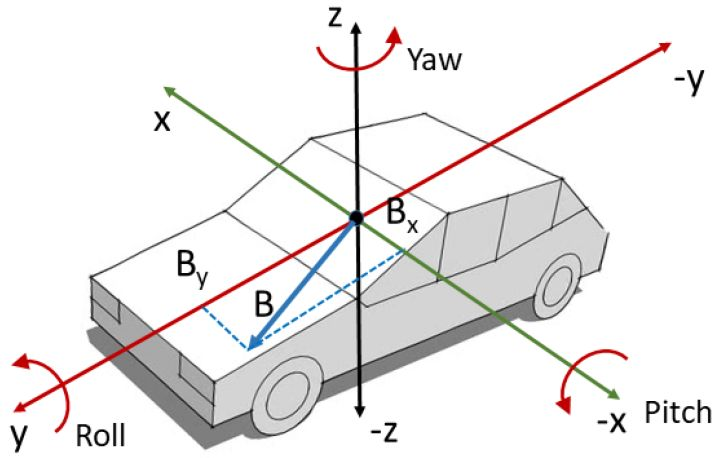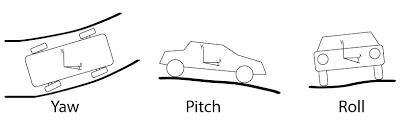### 相关定义

$$\mathbf{q}=[q_w\ q_x\ q_y\ q_z]$$

$$\boldsymbol{\omega}_t=[\omega_x\ \omega_y\ \omega_z]$$

### 四元素乘积的导数

$$\mathbf{q}(t+\Delta t)=\Delta \mathbf{q}\mathbf{q}(t)\tag{3-1}$$

$$\mathbf{u}=\frac{\mathbf{\omega}}{||\mathbf{\omega}||}$$
$$\theta=||\mathbf{\omega}||\Delta t$$

$$\begin{split} \Delta \mathbf{q}&=cos\frac{\theta}{2}+\mathbf{u}sin\frac{\theta}{2}\ &=cos\frac{||\omega||\Delta t}{2}+\frac{\omega}{||\omega||}sin\frac{||\omega||\Delta t}{2} \end{split}$$

$$\begin{split}\begin{array}{rcl} \mathbf{q}(t+\Delta t)-\mathbf{q}(t) &=& \Big(\cos\frac{|\boldsymbol\omega|\Delta t}{2} + \frac{\boldsymbol\omega}{|\boldsymbol\omega|}\sin\frac{|\boldsymbol\omega|\Delta t}{2}\Big)\mathbf{q} - \mathbf{q} \ &=& \Big(-2\sin^2\frac{|\boldsymbol\omega|\Delta t}{4} + \frac{\boldsymbol\omega}{|\boldsymbol\omega|}\sin\frac{|\boldsymbol\omega|\Delta t}{2}\Big)\mathbf{q} \end{array}\end{split}$$

$$\begin{split}\begin{array}{rcl} \dot{\mathbf{q}} &=& \underset{\Delta t\to 0}{\mathrm{lim}} \frac{\mathbf{q}(t+\Delta t)-\mathbf{q}(t)}{\Delta t} \ &=& \underset{\Delta t\to 0}{\mathrm{lim}} \frac{1}{\Delta t}\Big(-2\sin^2\frac{|\boldsymbol\omega|\Delta t}{4} + \frac{\boldsymbol\omega}{|\boldsymbol\omega|}\sin\frac{|\boldsymbol\omega|\Delta t}{2}\Big)\mathbf{q} \ &=& \frac{\boldsymbol\omega}{|\boldsymbol\omega|} \underset{\Delta t\to 0}{\mathrm{lim}} \frac{1}{\Delta t}\sin\big(\frac{|\boldsymbol\omega|\Delta t}{2}\big) \mathbf{q} \ &=& \frac{\boldsymbol\omega}{|\boldsymbol\omega|} \frac{d}{dt} \sin\big(\frac{|\boldsymbol\omega|}{2}t\big) \Big |_{t=0} \mathbf{q} \ &=& \frac{1}{2}\boldsymbol\omega \mathbf{q} \ &=& \frac{1}{2} \begin{bmatrix} -\omega_x q_x -\omega_y q_y - \omega_z q_z\ \omega_x q_w + \omega_z q_y - \omega_y q_z\ \omega_y q_w - \omega_z q_x + \omega_x q_z\ \omega_z q_w + \omega_y q_x - \omega_x q_y \end{bmatrix} \end{array}\end{split}$$

$$\begin{split}\boldsymbol\Omega(\boldsymbol\omega) = \begin{bmatrix} 0 & -\omega_x & -\omega_y & -\omega_z \ \omega_x & 0 & \omega_z & -\omega_y \ \omega_y & -\omega_z & 0 & \omega_x \ \omega_z & \omega_y & -\omega_x & 0 \end{bmatrix}\end{split}$$

$$\dot{\mathbf{q}} = \frac{1}{2}\boldsymbol\Omega(\boldsymbol\omega)\mathbf{q}\tag{3-2}$$

$$\mathbf{q}_{t+1} = \mathbf{q}_t + \dot{\mathbf{q}}_t\Delta t + \frac{1}{2!}\ddot{\mathbf{q}}t\Delta t^2 + \cdots$$

$$\mathbf{q} {t+1} = \Bigg[\mathbf{I}_4 + \frac{1}{2}\boldsymbol\Omega(\boldsymbol\omega)\Delta t\Bigg]\mathbf{q}_t\tag{3-3}$$

### 状态向量及控制输入

$$\mathbf{x}_t=\begin{bmatrix} q_w\ q_x\ q_y\ q_z \end{bmatrix}$$

$$\mathbf{u}_t=\begin{bmatrix} \omega_x\ \omega_y\ \omega_z \end{bmatrix}$$

### 状态方程及其雅各比矩阵

$$\begin{split} \mathbf{x}t&=\mathbf{f}(\mathbf{x}{t-1}, \mathbf{u}t) + \mathbf{w}=\Delta \mathbf{q}\mathbf{x}{t-1}+\mathbf{w} \end{split}$$

$$\begin{split} \mathbf{f}(\mathbf{x}_{t-1},\mathbf{u}t)&\approx \Bigg[\mathbf{I}4 + \frac{1}{2}\boldsymbol\Omega(\mathbf{u})\Delta t\Bigg]\mathbf{x}{t-1}\ &=\begin{bmatrix} q_w - \frac{\Delta t}{2} \omega_x q_x - \frac{\Delta t}{2} \omega_y q_y - \frac{\Delta t}{2} \omega_z q_z\ q_x + \frac{\Delta t}{2} \omega_x q_w - \frac{\Delta t}{2} \omega_y q_z + \frac{\Delta t}{2} \omega_z q_y\ q_y + \frac{\Delta t}{2} \omega_x q_z + \frac{\Delta t}{2} \omega_y q_w - \frac{\Delta t}{2} \omega_z q_x\ q_z - \frac{\Delta t}{2} \omega_x q_y + \frac{\Delta t}{2} \omega_y q_x + \frac{\Delta t}{2} \omega_z q_w \end{bmatrix}\ &\approx \mathbf{x} {t-1}+\mathbf{A}_t(\mathbf{x}t-\mathbf{x}{t-1}) \end{split}$$

$$\mathbf{A}t=\frac{\partial f}{\partial \mathbf{x}{t-1}}=\begin{bmatrix} 1 & - \frac{\Delta t}{2} \omega_x & - \frac{\Delta t}{2} \omega_y & - \frac{\Delta t}{2} \omega_z\ \frac{\Delta t}{2} \omega_x & 1 & \frac{\Delta t}{2} \omega_z & - \frac{\Delta t}{2} \omega_y\ \frac{\Delta t}{2} \omega_y & - \frac{\Delta t}{2} \omega_z & 1 & \frac{\Delta t}{2} \omega_x\ \frac{\Delta t}{2} \omega_z & \frac{\Delta t}{2} \omega_y & - \frac{\Delta t}{2} \omega_x & 1 \end{bmatrix}$$

### 预测方程

$$\hat{\mathbf{x}}_t^-=\Bigg[\mathbf{I}4 + \frac{1}{2}\boldsymbol\Omega(\mathbf{u})\Delta t\Bigg]\hat{\mathbf{x}}{t-1}^+\tag{3-4}$$
$$\mathbf{P}_t^- = \mathbf{A}t\mathbf{P}{t-1}^+\mathbf{A}_t^T + \mathbf{Q}_t\tag{3-5}$$
$$\mathbf{A}_t=\begin{bmatrix} 1 & - \frac{\Delta t}{2} \omega_x & - \frac{\Delta t}{2} \omega_y & - \frac{\Delta t}{2} \omega_z\ \frac{\Delta t}{2} \omega_x & 1 & \frac{\Delta t}{2} \omega_z & - \frac{\Delta t}{2} \omega_y\ \frac{\Delta t}{2} \omega_y & - \frac{\Delta t}{2} \omega_z & 1 & \frac{\Delta t}{2} \omega_x\ \frac{\Delta t}{2} \omega_z & \frac{\Delta t}{2} \omega_y & - \frac{\Delta t}{2} \omega_x & 1 \end{bmatrix}\tag{3-6}$$

### 测量更新

$$\hat{\mathbf{x}}_t^+=\hat{\mathbf{x}}_t^-+K_t\Big(\mathbf{z}_t-\mathbf{h}(\hat{\mathbf{x}}_t^-)\Big)$$

$$\mathbf{a}_t=\begin{bmatrix}a_x \ a_y \ a_z\end{bmatrix}$$

#### 测量模型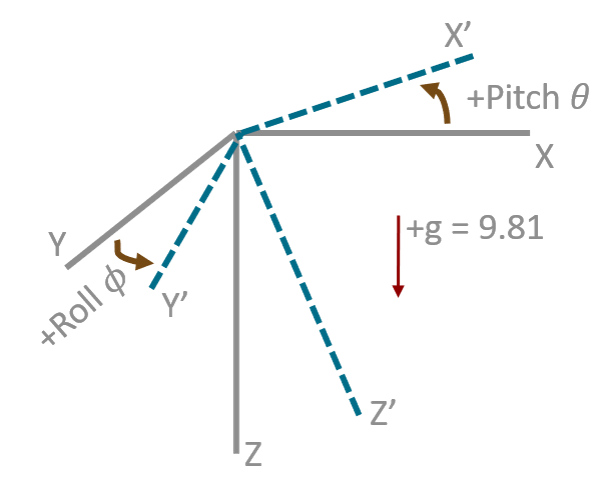$$\mathbf{g}=\mathbf{g}_{\mathrm{XYZ}}=[0\ 0\ 1]^T$$

$$\mathbf{g}^{\prime}=\mathbf{g}_{X^\prime Y^\prime Z^\prime}=\frac{\mathbf{a}_t}{||\mathbf{a}_t||}$$

$\mathbf{g}$和$\mathbf{g}^{\prime}$是重力加速度在两个不同坐标系下的表达，同时，这两个坐标系之间的旋转是此时的状态向量，因此
$$\mathbf{z}_t=\mathbf{h}(\mathbf{x}_t)=\mathbf{R}(\mathbf{x}_t)\mathbf{g}+\mathbf{v}_t\tag{3-7}$$

$$\mathbf{z}_t=\frac{\mathbf{a}_t}{||\mathbf{a}_t||}$$
$$Var(\mathbf{v}_t)=\mathbf{R}_t$$
$\mathbf{R}(\mathbf{q})$是将四元数转成旋转矩阵的表达，旋转矩阵左乘一个三位的列向量表示将该向量进行三维旋转。即下面的形式（$\mathbf{x}_t=[q_w\ q_x\ q_y\ q_z]$是我们之前定义过的）
$$\mathbf{R}(\mathbf{x}_t)=\begin{bmatrix} 1-2(q_y^2+q_z^2) & 2(q_xq_y-q_wq_z) & 2(q_xq_z+q_wq_y) \ 2(q_xq_y+q_wq_z) & 1-2(q_x^2+q_z^2) & 2(q_yq_z-q_wq_x) \ 2(q_xq_z-q_wq_y) & 2(q_wq_x+q_yq_z) & 1-2(q_x^2+q_y^2) \end{bmatrix}$$

#### 雅各比矩阵

$$\mathbf{h}(\mathbf{x}_t)=2 \begin{bmatrix} g_x (\frac{1}{2} - q_y^2 - q_z^2) + g_y (q_xq_y - q_wq_z) + g_z (q_xq_z + q_wq_y) \ g_x (q_xq_y + q_wq_z) + g_y (\frac{1}{2} - q_x^2 - q_z^2) + g_z (q_yq_z - q_wq_x) \ g_x (q_xq_z - q_wq_y) + g_y (q_wq_x + q_yq_z) + g_z (\frac{1}{2} - q_x^2 - q_y^2) \end{bmatrix}$$

$$\begin{split} \mathbf{H}(\mathbf{x}_t) &= \frac{\partial\mathbf{h}(\mathbf{x}_t)}{\partial\mathbf{x}_t} \ &=\begin{bmatrix} \frac{\partial\mathbf{h}(\mathbf{x}_t)}{\partial q_w} & \frac{\partial\mathbf{h}(\mathbf{x}_t)}{\partial q_x} & \frac{\partial\mathbf{h}(\mathbf{x}_t)}{\partial q_y} & \frac{\partial\mathbf{h}(\mathbf{x}_t)}{\partial q_z} \end{bmatrix} \ &= 2 \begin{bmatrix} -g_yq_z + g_zq_y & g_yq_y + g_zq_z & - 2g_xq_y + g_yq_x + g_zq_w & - 2g_xq_z - g_yq_w + g_zq_x \ g_xq_z - g_zq_x & g_xq_y - 2g_yq_x - g_zq_w & g_xq_x + g_zq_z & g_xq_w - 2g_yq_z + g_zq_y \ -g_xq_y + g_yq_x & g_xq_z + g_yq_w - 2g_zq_x & -g_xq_w + g_yq_z - 2g_zq_y & g_xq_x + g_yq_y \end{bmatrix}\end{split}$$

#### 更新方程

$$\hat{\mathbf{x}}_t^+=\hat{\mathbf{x}}_t^-+\mathbf{K}_t\Big(\mathbf{z}_t-\mathbf{h}(\hat{\mathbf{x}}_t^-)\Big)\tag{3-8}$$
$$\mathbf{K}_t=\mathbf{P}_t^-\mathbf{H}^T(\hat{\mathbf{x}}_t^-)\mathbf{S}_t^{-1}\tag{3-9}$$
$$\mathbf{S}_t=\mathbf{H}(\hat{\mathbf{x}}_t^-)\mathbf{P}_t^-\mathbf{H}^T(\hat{\mathbf{x}}_t^-)+\mathbf{R}_t\tag{3-10}$$

## 状态误差卡尔曼滤波器（ErKF : Error-state Kalman Filter)

### 概述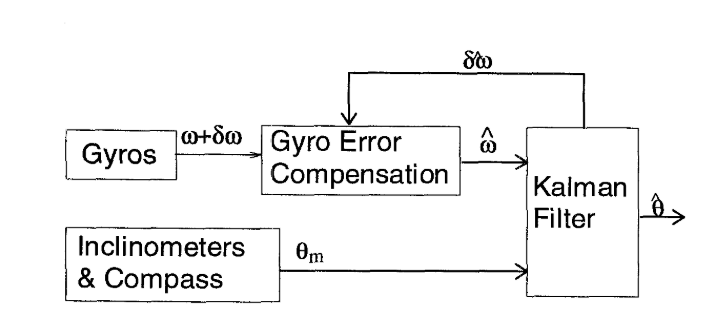PS: 要强调的是，各种卡尔曼滤波的形式多种多样，同时各种符号的定义也都并不完全一致，这也是入门卡尔曼滤波比较难的地方，有时候找资料都不知道怎么找。这也是写这篇文章的目的，提供一个基础的脉络给卡尔曼滤波的初学者。因此这里给出的ErKF只是形式之一，主要是引用自论文Extended Kalman Filter vs. Error State Kalman Filter for Aircraft Attitude Estimation

### 状态误差的递推公式

$$\dot{\mathbf{x}}(t)=\mathbf{f}\big(\mathbf{x}(t)\big)$$

$$\begin{split} \dot{\mathbf{x}}(t)+\delta \dot{\mathbf{x}}(t)&=\mathbf{f}\big(\mathbf{x}(t)+\delta \mathbf{x}(t)\big)\ &=\mathbf{f}(\mathbf{x}(t)) + \frac{\partial\mathbf{f}}{\partial \mathbf{x}(t)}\Big(\mathbf{x}(t)+\delta \mathbf{x}(t)-\mathbf{x}(t)\Big) + \boldsymbol{O}\ &\approx \mathbf{f}(\mathbf{x}(t)) + \frac{\partial\mathbf{f}}{\partial \mathbf{x}(t)}\delta \mathbf{x(t)} \end{split}$$

$$\delta \dot{\mathbf{x}}(t) = \frac{\partial\mathbf{f}}{\partial \mathbf{x}(t)}\delta \mathbf{x(t)}$$

$$\frac{\delta \mathbf{x}_{t+\Delta t} - \delta \mathbf{x}_t}{\Delta t} = \frac{\partial \mathbf{f}}{\partial \mathbf{x}_t}\delta \mathbf{x}t$$

$$\begin{split} \delta \mathbf{x} {t+1} &= \delta \mathbf{x}_t+\mathbf{A}_t\delta \mathbf{x}_t\Delta t\ &=\Big(\mathbf{I}+\mathbf{A}_t\Delta t\Big)\delta \mathbf{x}_t\ &=\Phi\delta \mathbf{x}_t \end{split}\tag{4-1}$$

### 预测过程

1. 在卡尔曼滤波系统外使用积分算出此时的系统状态
2. 使用卡尔曼滤波算出此时系统状态的误差
3. 将积分出来的系统状态弥补上卡尔曼滤波计算出误差

#### 系统状态计算

$$\hat{\mathbf{x}}{t}^-=\hat{\mathbf{x}}{t-1}^++\int^t_{t-\Delta t}\mathbf{f}\big(\mathbf{x}(t)\big)$$

#### 状态误差方程及预测方程

$$\delta \mathbf{x}_t=\Big(\mathbf{I}+\mathbf{A}t\Delta t\Big)\delta \mathbf{x}{t-1}+\mathbf{w}_t\tag{4-2}$$

$$\delta \hat{\mathbf{x}}_t^-=\Big(\mathbf{I}+\mathbf{A}t\Delta t\Big)\delta \hat{\mathbf{x}}^+{t-1}\tag{4-3}$$

$$P_t^-=\Phi_tP_{t-1}^+\Phi_t^T+Q_t\tag{4-4}$$

$$\Phi_t = \mathbf{I}+\mathbf{A}_t\Delta t$$

$$\mathbf{A}t = \frac{\partial f}{\partial \mathbf{x}}(\hat{\mathbf{x}}{t-1}^+)$$

#### 测量方程及更新方程

$$\mathbf{z}_t=\mathbf{h}(\mathbf{x}_t)+\mathbf{v}_t\tag{4-5}$$

1. 先更新对状态误差的估计
2. 更新状态的估计（即把状态误差弥补到$\hat{\mathbf{x}}_t^-$)
$$\delta \hat{\mathbf{x}}_t^+=K_t\big(\mathbf{z}_t-\mathbf{h}(\hat{\mathbf{x}}_t^-)\big)\tag{4-6}$$

$$K_t=P_t^-H_t^TS_t^{-1}\tag{4-7}$$

$$S_t=H_tP_t^-H_t^T+R_t\tag{4-8}$$

$$H_t = \frac{\partial \mathbf{h}}{\partial \mathbf{x}}(\hat{\mathbf{x}}_t^-)\tag{4-9}$$

$$\hat{\mathbf{x}}_t^+=\hat{\mathbf{x}}_t^-+\delta \hat{\mathbf{x}}_t^+\tag{4-10}$$

## 互补卡尔曼滤波

### 从加速度计计算姿态角roll、pitch$$Pitch=\theta = arcsin\frac{-a_x}{\sqrt{a_x^2+a_y^2+a_z^2}}=arcsin\frac{-a_x}{g}=arctan\frac{-a_x}{\sqrt{a_y^2+a_z^2}}$$

$$Roll=\phi=arctan\frac{a_y}{a_z}$$

$$g=9.81m/s^2$$

### 从磁力计计算姿态角yaw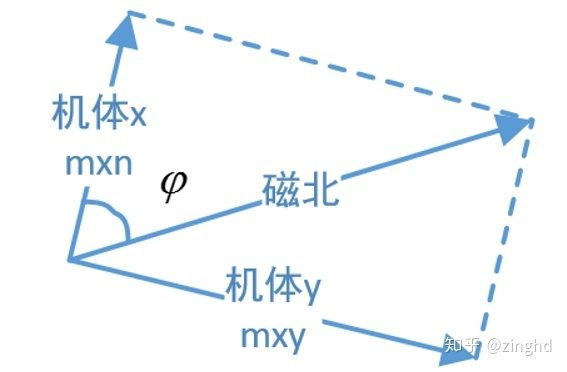### 从加速度计算的姿态弥补

1. 加速度计只能计算出Roll角和Pitch角，因此yaw角无法得到互补信息
2. 当车辆处于较大的加速度运动状态时，三轴加速度的合向量跟重力加速度会有偏差，因此互补结果应该根据这个偏差的大小做改变。

### 互补滤波器

$$\mathbf{q}=(1-\alpha)\mathbf{q}{\omega}+\alpha\mathbf{q}{am}$$

### 互补卡尔曼滤波器

1. 卡尔曼滤波器输出姿态角的误差和角速度的误差
2. 将当前时刻角速度的输出加上角速度的误差，并利用积分公式算出姿态角
3. 将步骤2算出的姿态角加上步骤1输出的姿态角误差

### 状态向量和测量向量

$$\delta \mathbf{x}_t= \begin{bmatrix} \delta \boldsymbol{\Theta}\ \delta \boldsymbol{\Omega} \end{bmatrix} =\begin{bmatrix} \delta \psi & \delta\theta & \delta\phi & \delta \omega_x & \delta \omega_y & \delta \omega_z \end{bmatrix}^T$$

$$\mathbf{z}_t= \begin{bmatrix} \psi_m-\hat{\psi} & \theta_m - \hat{\theta} & \phi_m - \hat{\phi} \end{bmatrix}^T$$

### 状态方程

$$\dot{\boldsymbol{\Theta}}(t)=\mathbf{f}\big(\mathbf{\Theta}(t),\mathbf{\Omega}(t)\big)$$

$$\delta \mathbf{\Theta}t=\delta \mathbf{\Theta}{t-1}+ \frac{\partial f}{\partial \mathbf{\Theta}}\delta \mathbf{\Theta}{t-1}+ \frac{\partial f}{\partial \mathbf{\Omega}}\delta \mathbf{\Omega} {t-1}$$

$$\delta \mathbf{\Omega}t = \delta \mathbf{\Omega}{t-1}$$

$$\delta \mathbf{x}_t= \begin{bmatrix} \delta \boldsymbol{\Theta}t\ \delta \boldsymbol{\Omega}t \end{bmatrix}= \begin{bmatrix} A_t& B_t\ \mathbf{0} & \mathbf{I} \end{bmatrix} \begin{bmatrix} \delta \boldsymbol{\Theta} {t-1}\ \delta \boldsymbol{\Omega} {t-1} \end{bmatrix}+\mathbf{w}_t\tag{5-1}$$

### 测量方程

$$\mathbf{z}t= \begin{bmatrix} \mathbf{I} {3x3} & \mathbf{0}\ \mathbf{0} & \mathbf{0}_{3x3} \end{bmatrix} \delta \mathbf{x}_t + \mathbf{v}_t\tag{5-2}$$

### 预测&&更新过程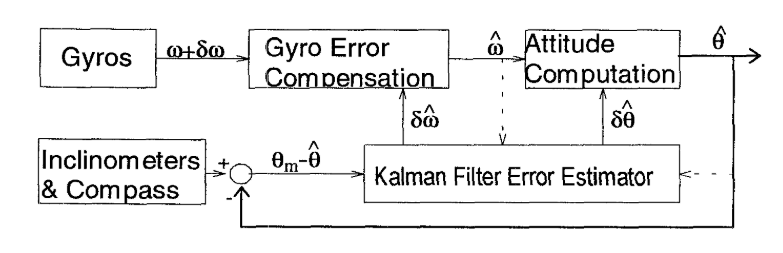## 后话

1. 卡尔曼滤波是一个很古老的算法，但同时又是被广泛应用的算法。即使在今天姿态解算中很多用了因子图，但是对IMU的预积分依旧要使用卡尔曼滤波。但是卡尔曼滤波算法只是一个工具，不同的系统建模方式会产生不同形式的卡尔曼滤波器，这也导致了初学者不知道从哪里入手。
2. 在查资料的过程中发现，卡尔曼滤波的一些变种如Error-State Kalman Filter和Complimentary Kalman Filter其实并不是严格定义的。
3. 笔者对卡尔曼滤波并没有很丰富的应用经验，本身也不是专门做运动控制和姿态解算的。本文的叙述在追求通俗易懂之外尽力保证公式和符号定义的准确。但无法保证没有错误。
4. 对于卡尔曼滤波器中各个变量的初始值设置是门学问，论文中都会有独立的章节讲述初始值如何设置。这方面可能得结合实际应用和效果得出最优的方案。

## Reference

 四元素乘积求导